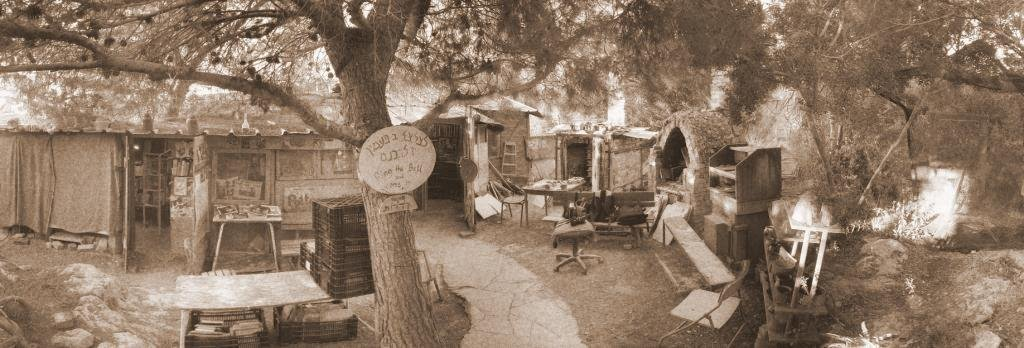## Saturday, June 27, 2009

### Odious Number: 69

The aliquot sum of sixty-nine is 27 within the aliquot sequence (69,27,13,1,0) 69 being the third composite number in the 13-aliquot tree. 69 is a semiprime. Furthermore, since the two factors of 69 are both Gaussian primes, 69 is a Blum integer. Adding up the divisors of 1 through 9 gives 69. Because 69 has an odd number of 1s in its binary representation, it is sometimes called an "odious number." Of note is that 69² (4 761) and 69³ (328 509) uses every digit from 0-9. 69 is equal to 105 octal, while 105 is equal to 69 hexadecimal. This same property can be applied to all numbers from 64 to 69. On many handheld scientific and graphing calculators, the highest factorial that can be computed within memory limitations is 69! or 1.711224524*1098. The number 69 can be rotated 180° and remain the same...